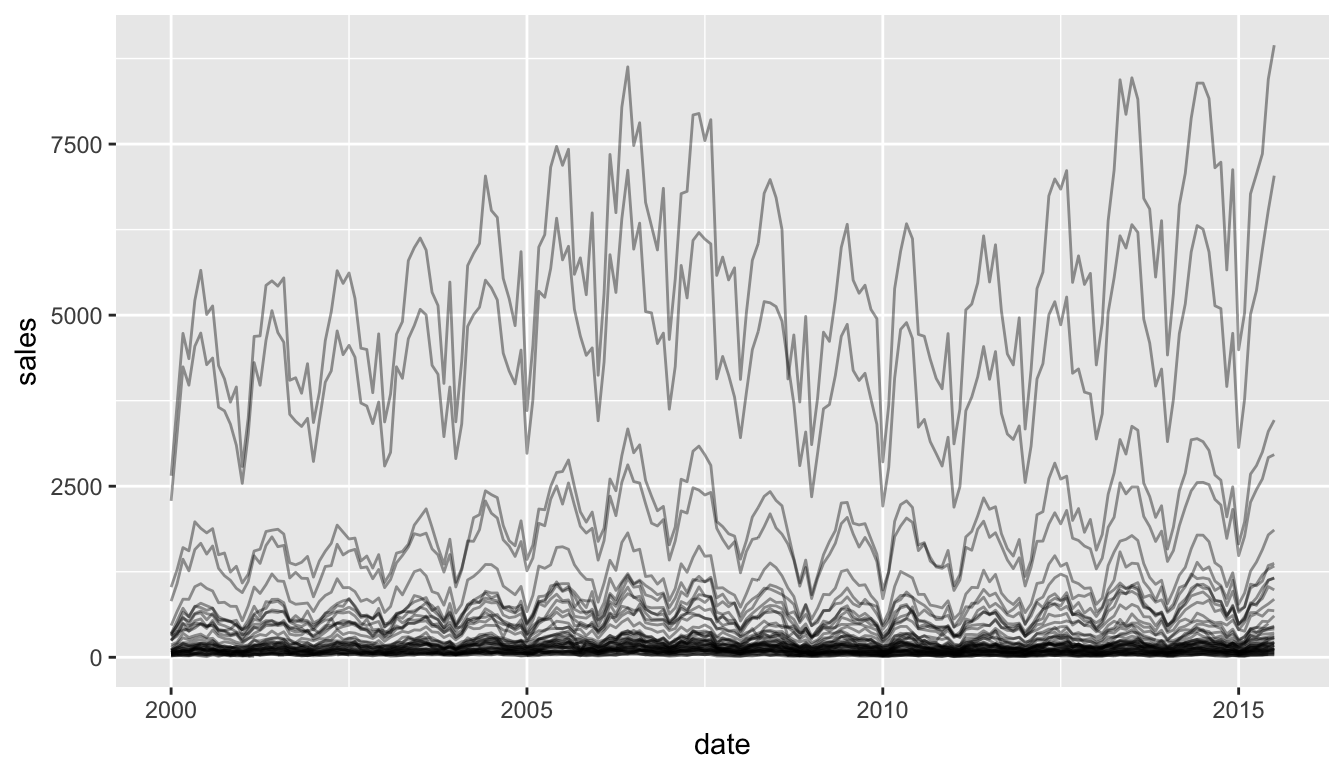4/7/2017

## Examples on the web

Some super cool examples include:

## Main tools in R

Make your scatter plots, line plots, bar plots, etc interactive

• Plotly

• Highcharts

• crosstalk

Make D3.js plots in R (no javascript required!)

• rCharts (D3.js)

• d3scatter

• networkD3

## ggplot2

```library(ggplot2)
g <- ggplot(txhousing, aes(x = date, y = sales, group = city)) +
geom_line(alpha = 0.4)
g```## ggplot2 + plotly

```library(plotly)
g <- ggplot(txhousing, aes(x = date, y = sales, group = city)) +
geom_line(alpha = 0.4)
ggplotly(g, tooltip = c("city"))```

## plotly

```g <- txhousing %>%
# group by city
group_by(city) %>%
# initiate a plotly object with date on x and median on y
plot_ly(x = ~date, y = ~median) %>%
# add a line plot for all texan cities
add_lines(name = "Texan Cities", hoverinfo = "none",
type = "scatter", mode = "lines",
line = list(color = 'rgba(192,192,192,0.4)')) %>%
# plot separate lines for Dallas and Houston
`g %>% rangeslider`